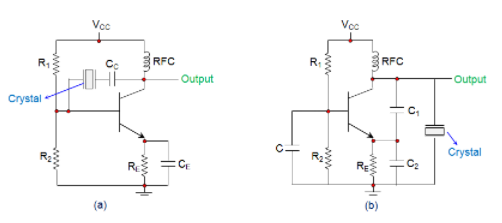Goseeko blog# What is a Crystal Oscillator?

Crystal Oscillator is specially designed in a circuit to provide low impedance when it operates in series-resonant mode and high impedance. It is then achieved by connecting a crystal into the circuit. The circuit when operated in series resonant mode (figure a) and anti-resonant mode (figure b) is below .## Working

In the circuits shown, the resistor R1 and R2 form the voltage divider network while the emitter resistor RE stabilizes the circuit. Further, CE (Figure a) acts as an AC bypass capacitor while the coupling capacitor CC (Figure a) is there to block DC signal propagation between the collector and the base terminals. Next, the capacitors C1 and C2 form the capacitive voltage divider network in the case of Figure b.

In both the above circuits we can see there is a Radio Frequency Coil (RFC). This RFC then offers two advantages. There is no affect as it frees the output of the circuit from the AC signal present on the power line. Secondly, it also provides DC bias to the circuit.

The amplitude of the oscillations increases to an extent till the point comes when the loop gain reduces to unity. This is because of the nonlinearities in the amplifier. The frequency of the operating circuit is affecting because of the feedback loop of the crystal when the oscillator reaches the steady state.

To fulfil the Barkhausen Criterion the crystal frequency self-adjusts and present a reactance to the circuit. The crystal’s fundamental or characteristic frequency, which we decide by the physical size and shape of the crystal, fixes the frequency of the crystal oscillator.

There may be multiple frequencies or harmonics in the crystal. This may happen if the crystal is non-parallel or it has non-uniform thickness. Also the tuning of the crystal oscillator  for odd or even harmonics of fundamental frequencies can be done. These are harmonics and overtone oscillators respectively.

Interested in learning about similar topics? Here are a few hand-picked blogs for you!